• 能量信号
2022-02-28 19:38:02

### 文章目录

信号根据 " 能量 " 可以分为 " 能量信号 "" 功率信号 " ;

• 信号能量定义 : 整个轴上的能量先进行平方 , 然后求积分 ; 如果 能量 小于 无穷 , 则该信号 是 能量信号 ; 有限区间内的信号称为能量信号 ;

• 信号功率定义 : 在一个信号周期内 , 进行积分求和操作 ; 如果 功率 小于 无穷 , 则该信号 是 功率信号 ; 周期信号 , 随机信号 是功率信号 ;

本篇博客中的 互相关函数自相关函数 , 都是 " 能量信号 "相关函数 ;

# 一、互相关函数

互相关函数 表示的是 两个不同的信号 之间的相关性 ;

x ( n ) x(n) y ( n ) y(n) " 互相关函数 " 如下 ,

r x y ( m ) = ∑ n = − ∞ + ∞ x ∗ ( n ) y ( n + m ) r_{xy}(m) = \sum_{n=-\infty}^{+\infty} x^*(n) y(n + m)

其中 y ( n ) y(n) 进行了移位 , 向左移动了 m m 单位 ,

该 " 互相关函数 " 求的是 y ( n ) y(n) 移位 m m 后的序列 x ( n ) x(n) 序列之间的关系 ;

注意这里的 n n 表示的是时刻 , m m 表示的是信号移动的间隔 ;

" 互相关函数 " 表示的是 x ( n ) x(n) 信号 , 与 隔了 m m 时间后的 y ( n ) y(n) 信号之间的关系 ;

2 2 个信号 ( 序列 ) 之间 " 关系 " 是一个 函数 , 函数的自变量是 m m 间隔 , 不是 n n ;

# 二、自相关函数

自相关函数 ( Autocorrelation Function ) :

r x x ( m ) = ∑ n = − ∞ + ∞ x ∗ ( n ) x ( n + m ) = r x ( m ) r_{xx}(m) = \sum_{n=-\infty}^{+\infty} x^*(n) x(n + m) = r_x(m)

" 自相关函数 " 是 " 自己信号 " 与 " 隔一段时间后的 自己信号 " 之间的 相关性 ;

如果 m = 0 m = 0 时 , " 自己信号 " 与 " 隔一段时间 m m 后的自己信号 " 完全相等 , 该值就是 信号的能量 ;

r x ( 0 ) = ∑ n = − ∞ + ∞ ∣ x ( n ) ∣ 2 = E r_{x}(0) = \sum_{n=-\infty}^{+\infty} |x(n)|^2= E数字信号处理 相关函数 互相关函数 自相关函数
更多相关内容
• 在激光惯性约束聚变(ICF)受激散射光能量信号的检测中，背景噪声的存在严重影响了有用信息的提取。针对该问题，设计了一种新型的可变参数放大滤波电路(VPAFC)，并在降噪源分离(DSS)技术的基础上提出了一种信号降噪...
• 能量有限，功率必然为0，称为能量信号； 能量无穷，功率有限，称为功率信号； 存在能量无限且功率无限的信号。 能量信号和功率信号的举例 Ans:功率信号 Ans：能量信号 Ans：非能量信号非功率信号 ...

### 信号的能量与功率

功率和能量类似于密度和质量的关系。

连续信号的能量是积分形式，，用极限形式表示为

离散信号的能量是求和形式，，用极限形式表示为

功率是能量在时间上的平均

连续信号的功率为

离散信号的功率为

### 能量信号和功率信号的定义

能量有限，功率必然为0，称为能量信号

能量无穷，功率有限，称为功率信号

存在能量无限且功率无限的信号。

### 能量信号和功率信号的举例

Ans:功率信号

Ans：能量信号

Ans：非能量信号非功率信号

（洛必达法则或者泰勒级数展开求极限）

Ans： 功率信号

总结：在无限时间的周期信号为功率信号；能量信号一定是非周期信号

展开全文• ## 能量信号和功率信号的分别

万次阅读 多人点赞 2019-09-22 12:38:02
判断一个信号是能量信号还是功率信号，首先需要计算其能量和功率。 不想看公式的可以直接跳到加粗的结论部分。 对于信号f(t)，其能量为： f(t)信号的能量 其功率为： f(t)信号的功率 为什么要这样定义呢？...

首先要明确一点，这两种信号概念是建立在无穷大的时间积分的基础上的。

一.能量与功率

判断一个信号是能量信号还是功率信号，首先需要计算其能量和功率。

不想看公式的可以直接跳到加粗的结论部分。

对于信号f(t)，其能量为：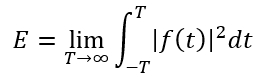f(t)信号的能量

其功率为：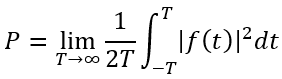f(t)信号的功率

为什么要这样定义呢？

可以借助物理上的概念辅助理解一下。对于电阻R，施加电压f(t)，在区间(-∞,+∞)上，其能量就是：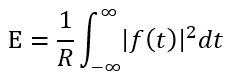式中的R为常数，抛弃物理场景后，取R的值为1，就能得到能量的表达式。

能量的公式建立有功率就很容易了，就是能量除以时间。（想想家里的电灯泡）

换句话说：

能量就是信号的平方在区间(-∞,+∞)上的积分。

功率就是能量与“无穷长的时间”的比值。（该表达不严谨，只辅助理解）

二.能量信号与功率信号

知道能量和功率的概念之后，就会发现有三种组合存在：

有限能量+零功率

无穷能量+有限功率

无穷能量+无穷功率

（不要问为什么没有“零能量+有限功率”、“有限能量+无穷功率”等组合，因为“能量=功率*无穷时间”，所以能量是不会比功率“小”的）

这三种组合分别代表三种信号：

①有限能量+零功率 ----->能量信号

代表波形一：一个孤零零的方波。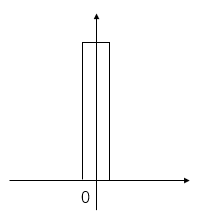代表波形二：一个极限值为0的波形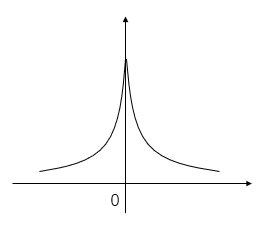②无穷能量+有限功率 ----->功率信号

代表波形一：一个无限延伸的正弦波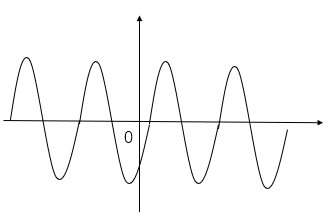代表波形二：无限长的白噪声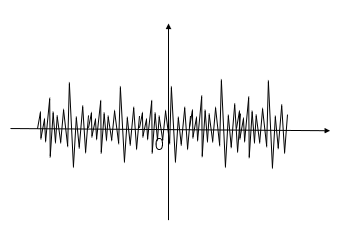③无穷能量+无穷功率----->非功非能信号

代表波形：一个无限延伸的单调波形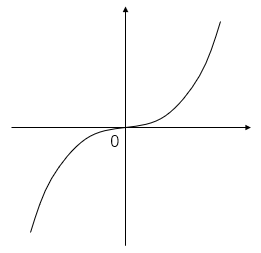概括一下：

能量有限、功率为零的信号为能量信号。

能量无限、功率有限的信号为功率信号。

所有周期信号都是功率信号；所有有限数量的脉冲信号都是能量信号。

那么问题来了：我用温度计采集了一堆温度值，这算什么信号呢？

个人认为属于功率信号，因为现实世界不可能在无限时间范围内采集信号。（如有更合适的答案或者解释欢迎指正）

文章如要转载请私信与我联系，并注明来源知乎专栏与信号处理有关的那些东东作者Mr.括号。

欢迎关注我的公众号“看海的城堡”，微信号为“khscience”，会有更多有趣的东西分享。

参考：

Energy (signal processing)

【图文】功率信号和能量信号专题_百度文库

展开全文功率信号
• 能量信号、功率信号及能量谱、功率谱能量信号与功率信号能量谱与功率谱 能量信号与功率信号 要区分功率谱和能量谱，首先要清楚两种不同类型的信号：功率信号和能量信号。我们从一个具体的物理系统来引出能量信号和...

# 能量信号与功率信号

要区分功率谱和能量谱，首先要清楚两种不同类型的信号：功率信号和能量信号。我们从一个具体的物理系统来引出能量信号和功率信号的概念。已知阻值为R的电阻上的电压和电流分别为v(t) 和 i(t)，则此电信号的瞬时功率为： p(t) = v2(t)/R = i2(t)R。在作定性分析时，为了方便起见，通常假设电阻R为1欧姆而得到归一化 (Normolized) 的功率值。作定量计算时可以通过去归一化，即将实际的电阻值代入即可得到实际的功率值。将上面的概念做一个抽象，对信号 x(t) 定义其瞬时功率为 |x (t)|2 ，在时间间隔 (-T/2 T/2) 内的能量为:
E x = ∫ − T / 2 T / 2 ∣ x ( t ) ∣ 2 d t   … … … （ 1 ） E_x= \int_{-T/2}^{T/2}| x(t)|^2dt\, ……… （1）
该间隔内的平均功率为：

p = E/T ……………………(2)

当且仅当f(t)在所有时间上的能量不为0且有限时，该信号为能量信号，即(1)式中的 T 趋于无穷大的时候E为有限。典型的能量信号如方波信号、三角波信号等。但是有些信号不满足能量信号的条件，如周期信号和能量无限的随机信号，此时就需要用功率来描述这类信号。当且仅当x(t)在所有时间上的功率不为0且有限时，该信号为功率信号，即 (2) 式中的 T 趋于无穷大的时候 p 为有限。系统中的波形要么具有能量值，要么具有功率值，因为能量有限的信号功率为0，而功率有限的信号能量为无穷大。一般来说，周期信号和随机信号是功率信号，而非周期的确定信号是能量信号。将信号区分为能量信号和功率信号可以简化对各种信号和噪声的数学分析。还有一类信号其功率和能量都是无限的，如 f(t) = t，这类信号很少会用到。

信号可以分成能量信号与功率信号，非周期能量信号具有能量谱密度，是傅立叶变换的平方，功率信号具有功率谱密度，其与自相关函数是一对傅立叶变换对，等于傅立叶变换的平方/区间长度。不能混淆。能量信号是没有功率谱的。

随机信号在时间上是无限的，在样本上也是无穷多，因此随机信号的能量是无限的，它应是功率信号。功率信号不满足付里叶变换的绝对可积的条件，因此其付里叶变换是不存在的。如确定性的正弦函数的付里叶变换是不存在，只有引入了冲激函数才求得其付里叶变换。因此，对随机信号的频谱分析，不再简单的是频谱，而是功率谱。

周期信号是功率信号，但是周期信号可能是确定性信号，也可能是随机信号，但是周期信号是存在功率谱密度的。对于持续时间无限长的随机信号来说，也是存在功率谱密度的。

一般来讲，对于随机信号，由于持续期时间无限长，不满足绝对可积与能量可积的条件，因此不存在傅立叶变换，所以我们只能研究其功率谱，因为样本函数的功率毕竟是有限哦。

对于确定性信号而言，里面存在能量信号，是没有功率谱密度的，也存在功率信号，是有功率谱密度的。所以信号的频谱与是否是确定性信号没有必然联系。

# 能量谱与功率谱

了解信号可能是能量信号，也可能是功率信号后，就可以很好地理解功率谱和能量谱的概念。对于能量信号，常用能量谱来描述。所谓的能量谱，也称为能量谱密度，是指用密度的概念表示信号能量在各频率点的分布情况。也即是说，对能量谱在频域上积分就可以得到信号的能量。能量谱是信号幅度谱的模的平方，其量纲是焦/赫。对于功率信号，常用功率谱来描述。所谓的功率谱，也称为功率谱密度，是指用密度的概念表示信号功率在各频率点的分布情况。也就是说，对功率谱在频域上积分就可以得到信号的功率。从理论上来说，功率谱是信号自相关函数的傅里叶变换。因为功率信号不满足傅里叶变换的条件，其频谱通常不存在，维纳-辛钦定理证明了自相关函数和傅里叶变换之间对应关系。在工程实际中，即便是功率信号，由于持续的时间有限，可以直接对信号进行傅里叶变换，然后对得到的幅度谱的模求平方，再除以持续时间来估计信号的功率谱。

对确定性的信号，特别是非周期的确定性信号，常用能量谱来描述。而对于随机信号，由于持续期时间无限长，不满足绝对可积与能量可积的条件，因此不存在傅立叶变换，所以通常用功率谱来描述。周期性的信号，也同样是不满足傅里叶变换的条件，常用功率谱来描述，这些在前面已经有所说明。只有如单频正弦信号等很少的特殊的信号，在引入delta函数之后，才可以求解信号的傅里叶变换。

对于用功率谱描述的随机信号而言，白噪声是一个特例。根据定义，白噪声是指功率谱密度在整个频域内均匀分布的噪声。严格地说，白噪声只是一种理想化模型，因为实际噪声的功率谱密度不可能具有无限宽的带宽，否则它的功率将是无限大，是物理上不可实现的。然而，白噪声在数学处理上比较方便，因此它是系统分析的有力工具。一般，只要一个噪声过程所具有的频谱宽度远远大于它所作用系统的带宽，并且在该带宽中其频谱密度基本上可以作为常数来考虑，就可以把它作为白噪声来处理。例如，热噪声和散弹噪声在很宽的频率范围内具有均匀的功率谱密度，通常可以认为它们是白噪声。

# 信号能量

由时域求能量：
E x = ∫ − ∞ ∞ ∣ x ( t ) ∣ 2 d t   … … … … … … （ 3 ） E_x= \int_{-\infty}^\infty| x(t)|^2dt\, ……………… （3）
根据傅立叶逆转换公式
x ( t ) = ∫ − ∞ ∞ X ( f ) e j 2 π f t d f   … … … （ 4 ） x(t)= \int_{-\infty}^\infty X(f)e^{j2\pi ft}df\, ……… （4）
将(4)代入(3)
∫ − ∞ ∞ [ ∫ − ∞ ∞ X ( f ) e j 2 π f t d f ] x ∗ ( t ) d t = ∫ − ∞ ∞ X ( f ) [ ∫ − ∞ ∞ x ∗ ( t ) e j 2 π f t d t ] d f = ∫ − ∞ ∞ X ( f ) X ∗ ( f ) d f = ∫ − ∞ ∞ ∣ X ( f ) ∣ 2 d f   … … … （ 5 ） \int_{-\infty}^\infty [\int_{-\infty}^\infty X(f)e^{j2\pi ft}df]x^\ast (t)dt=\int_{-\infty}^\infty X(f) [\int_{-\infty}^\infty x^*(t)e^{j2\pi ft}dt]df=\int_{-\infty}^\infty X(f)X^*(f)df=\int_{-\infty}^\infty |X(f)|^2df\, ……… （5）
故信号的能量(帕斯瓦尔定理）
E x = ∫ − ∞ ∞ ∣ x ( t ) ∣ 2 d t = ∫ − ∞ ∞ ∣ X ( f ) ∣ 2 d f   … … … （ 6 ） E_x=\int_{-\infty}^\infty| x(t)|^2dt=\int_{-\infty}^\infty |X(f)|^2df\, ……… （6）

## 能量谱密度

G x ( f ) ≡ ∣ X ( f ) ∣ 2 G_x(f) \equiv |X(f)|^2

## 能量信号自相关函数

信号 x(t) 的自相关函数
r x ( τ ) ≡ ∫ − ∞ ∞ x ( t ) x ∗ ( t + τ ) d t = x ( τ ) ∗ x ∗ ( − τ ) … … （ 7 ） r_x(\tau)\equiv \int_{-\infty}^{\infty}x(t)x^\ast(t+\tau)dt=x(\tau)*x^*(-\tau) ……（7）
x(t)的自相关函数取傅立叶转换可得x(t)的能量密度频谱
r x ( 0 ) = ∫ − ∞ ∞ x ( t ) x ∗ ( t ) d t = ∫ − ∞ ∞ ∣ x ( t ) ∣ 2 d t = E x … … （ 7 ） r_x(0)=\int_{-\infty}^{\infty}x(t)x^\ast(t)dt=\int_{-\infty}^{\infty}|x(t)|^2dt=E_x ……（7）

本文根据以下文章改写，谨表示感谢！
链接：

1. 与频谱有关的几个谱的概念 ：https://blog.csdn.net/iteye_9380/article/details/82136131
2. 信号的功率谱、能量谱、频谱的区别（转）：https://www.cnblogs.com/stucp/archive/2012/08/27/2658243.html
展开全文• 今天重新翻了翻通信原理课本，发现以前学习效率为什么低，就拿能量信号和功率信号来说 课本上，你会看到两者的定义公式，还有特性（诸如功率能量无穷功率不为零，能量信号能量一定，功率为零等等），看到这里好像很...经验分享
• 1. 周期信号 对于连续信号，若存在T>0T>0T>0，使 x(t)=x(t+nT),n为整数 x(t)=x(t+nT), \quad n 为整数 x(t)=x(t+nT),n为整数 对于离散信号，若存在大于零的整数N，使 x(n)=x(n+kN),k为整数 x(n)=x(n+kN), \...
• 能量信号为功率信号的积分，功率信号为能量信号的密度。对于一个信号，y=f(x)，能量相当于曲线和x轴的积分面积，功率相当于y值。当一个信号的积分面积无穷大，但是能找到一个不是无穷大的平均y值，那么这就是功率...
• 能量信号和功率信号 信号能量公式： 信号平均功率定义： 能量信号： 功率信号： 不存在既是能量信号也是功率信号的信号，但存在既不是能量信号也不是功率信号的信号 能量谱密度 S(f)是信号s(t)的傅里叶变换也...matlab
• 一、周期信号的频谱 1.1周期信号的虚指数/三角傅里叶级数： 其中， 1.2 意义：从频域上看，周期信号可以由其所有频率分量叠加而成，并且这些频域分量都是周期信号频率的整数倍，傅里叶级数的作用就是算出这些...matlab
• 总述 一、区分信号类型 根据式E=∫−∞∞s2(t)dtE=\int_{-\infty }^{\infty }...能量信号：能量有限，平均功率为0； 二、功率信号的分析 频谱（离散）：C(nf0)=1T0∫−T/2T/2s(t)e−j2πnf0tdtC\left( n{{f}_{0}} \r
• 既不是功率信号又不是能量信号：例如x=e^-t、x=t 无穷大 无穷大 能量信号:仅在有限区间内不为0的信号 有限 有限=0 功率信号：直流信号、周期信号、阶跃信号 无限 有限 不存在这种信号 ...
• ## 能量信号与功率信号

万次阅读 多人点赞 2018-06-23 21:31:33
信号的功率：归一化功率定义为：信号在单位电阻上消耗的功率。...如果上述积分存在，则称之为能量信号；积分不存在，如下：定义：这种能量无限，但平均功率有限的信号称为功率信号，通常，周期信号都是功率信号。...
• 一般来说，周期信号和随机信号是功率信号，而非周期的确定信号是能量信号。 注：随机信号只能用功率谱来描述它的频率特性。由于，无法用确定的时间函数表示，也就无法得到信号是频谱。 能量信号 　一个...
•  通常情况下，电信号默认为电流（I）或电压（V）,有两种主要类型：能量信号、功率信号。相信有朋友现在依然还是傻傻分不清楚这两者之间的区别。 下面我将进行分条详述：（关键词已加黑）  1）能量信号：表现为 ...
• 一、能量信号和功率信号  根据信号可以用能量式或功率式表示可分为能量信号和功率信号。 能量信号，如各类瞬变信号。 在非电量测量中，常将被测信号转换为电压或电流信号来处理。显然，电压信号加在单位...
• ## 信号能量与功率

千次阅读 2021-07-12 12:38:10
1.1.2 信号能量与功率 注意：信号在后面的表示和处理中，使用复数将会更方便，也能展现更多信息 (一)有限区间能量 对于一个连续时间信号x(t)x(t)x(t)来说，在t1≤t≤t2t_1\le t\le t_2t1​≤t≤t2​内的总能量可以...数学 傅立叶分析
• 这是我在知乎上的一个回答，鉴于很多朋友对这几个概念不是...信号按能量是否有限，可以分为：能量信号和功率信号，能量信号的能量是有限的，功率信号的能量是无限的。下面我们具体解释一下这两个概念。  在信号系...
• 代码演示说明了脑电信号频谱、能量、功率谱密度等相关联系，详见https://www.xxy.ink/learn/bci/4.html
• 对时序信号进行小波包分解，并求其每个子频带的能量占比。
• 该文件给出了计算信号能量的具体方法，matlab编程计算简单，速度快，通俗易懂，适合新手，将信号能量进行累加，便有观察检测
• 能量谱分析计算,人脸识别中的光照处理方法，分析了该信号的时域、频域、倒谱，循环谱等。
• 1.信号有能量信号和功率信号之分。 （1）信号的能量指的是信号电压或者电流f(t)加到1Ω电阻上所消耗的能量。 （2）信号的功率指的是信号电压或者电流f(t)在1Ω电阻上所消耗的功率。 能量信号指的是能量有限，功率为...
• 针对感应耦合电能传输(ICPT)系统电能与信号同步传输方法中信号反向传输的优化问题,在深入分析已有文献的基础上,采用信号并联注入、串联拾取的能量信号反向同步传输拓扑,并提出其参数设计方法。该拓扑充分将ICPT系统...
• 电子通信专业的信号检测当中的能量检测MATLAB信号仿真
• 利用matlab对语音信号短时时域处理，计算信号短时能量和短时平均幅度
• 能量信号：W为有限值，P为0（P为W与趋于无穷大的时间的比值，即为0）。 功率信号：P为有限值，W为无穷大（W为P与趋于无穷大的时间的乘机）。 一般的周期信号为功率信号；非周期信号在有限区间有值，为能量信号。 ......